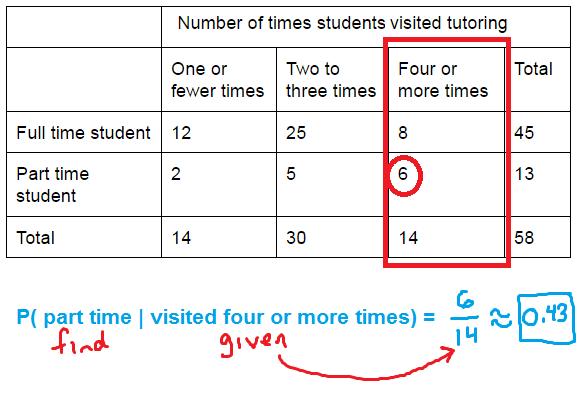# Conditional probability – notation and calculation

When finding a conditional probability, you are finding the probability that an event A will occur, given that another event, event B, has occurred. In this article, we will look at the notation for conditional probability and how to find conditional probabilities with a table or with a formula.

## Notation

The image below shows the common notation for conditional probability. You can think of the line as representing “given”. On the left is the event of interest, and on the right is the event we are assuming has occurred.With this notation, you could also use words to describe the events. For example, let’s say you wanted to find the probability someone buys a new car, when you know they have started a new job. This would be represented as:## Example using a table of data

One of the common types of problems you will see uses a two-way table of data. Here, we will look at how to find different probabilities using such a table.

### Example

A survey asked full time and part time students how often they had visited the college’s tutoring center in the last month. The results are shown below.Suppose that a surveyed student is randomly selected.

(a) What is the probability the student visited the tutoring center four or more times, given that the student is full time?

Conditional probability is all about focusing on the information you know. When calculating this probability, we are given that the student is full time. Therefore, we should only look at full time students to find the probability.(b) Suppose that a student is part time. What is the probability that the student visited the tutoring center one or fewer times?

This one is a bit more tricky due to the wording. Think of it in the following way:

• Find: probability student visited the tutoring center one or fewer times
• Assume or given: student is part time (“suppose that a student is part time”)

Since we are assuming (or supposing) the student is part time, we will only look at part time students for this calculation.(c) If the student visited the tutoring center four or more times, what is the probability he or she is part time?

As above, we need to make sure we know what is given, and what we are finding.

• Find: probability he or she is part time
• Assume or given: student visited the tutoring center four or more times (“if the student visited the tutoring center four or more times…”)

For this question, we are only looking at students who visited the tutoring center four or more times.As you can see, when using a table, you just need to pay attention to which group from the table you should focus on.

## Examples of using the formula to find conditional probability

In some situations, you will need to use the following formula to find a conditional probability.This formula could actually be used with the table data, though it is often easier to apply in problems similar to the next example.

### Example

In a sample of 40 vehicles, 18 are red, 6 are trucks, and 2 are both. Suppose that a randomly selected vehicle is red. What is the probability it is a truck?

We are asked to find the following probability:

$$\text{P(truck}|\text{red)}$$

Applying the formula:

\begin{align}\text{P(truck}|\text{red)} &= \dfrac{\text{P(truck and red)}}{\text{P(red)}}\\ &= \dfrac{\tfrac{2}{40}}{\tfrac{18}{40}}\\ &= \dfrac{2}{18}\\ &= \dfrac{1}{9} \approx \boxed{0.11}\end{align}

The thinking behind the formula is very similar to the thinking used with the table. For example, notice that what we “know” ends up on the bottom of the fraction. We can also apply this to situations where we are given probabilities and not counts.

### Example

A board game comes with a special deck of cards, some of which are black, and some of which are gold. If a card is randomly selected, the probability it is gold is 0.20, while the probability it gives a second turn is 0.16. Finally, the probability that it is gold and gives a second turn is 0.08.

Suppose that a card is randomly selected, and it allows a player a second turn. What is the probability it was a gold card?

This time, we are given the following probabilities:

• “the probability it is gold is 0.20” -> P(gold) = 0.2
• “the probability it gives a second turn is 0.16” -> P(second turn) = 0.16
• “the probability that it is gold and gives a second turn is 0.08” -> P(gold and second turn) = 0.08

We are trying to calculate:

$$\text{P(gold}|\text{second turn)}$$

We can apply the formula to find this probability:

\begin{align}\text{P(gold}|\text{second turn)} &= \dfrac{\text{P(gold and second turn)}}{\text{P(second turn)}}\\ &= \dfrac{0.08}{0.16}\\ &= \boxed{0.5}\end{align}

You can see that this works out very nicely if you take a moment to write down the information given in the problem. In fact, you could really say that about any real-life/ word problem in math!